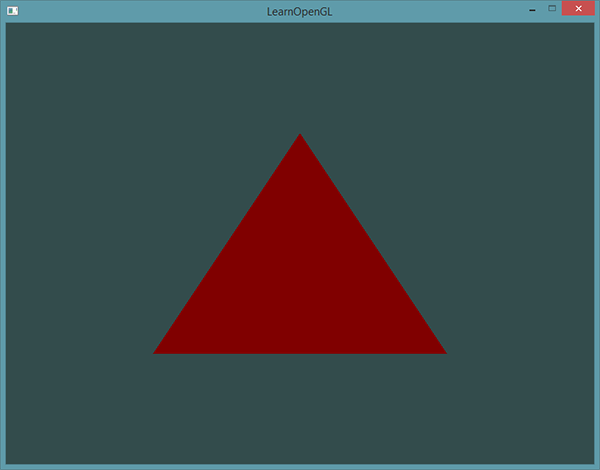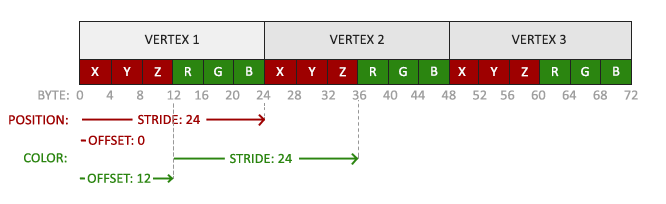• May, 05 2019
• OpenGL

# 着色器

## 着色器

### GLSL

``````#version version_number
in type invariable_name;
in type in_variable_name;

out type out_variable_name;

uniform type uniform_name;

int main(){
// 处理输入并进行一些图形操作
...
// 输出处理过的结果到输出变量
out_variable_name = weird_stuff_we_processed;
}``````

``````int nrAttributes;
glGetIntegerv(GL_MAX_VERTEX_ATTRIBS,&nrAttributes);
std::cout << "Maximum nr of vertex attributes supported: " << nrAttributes << std::endl;``````

### 数据类型

#### 向量

GLSL中的向量是一个可以包含有1、2、3或者4个分量的容器，分量的类型可以是前面默认基础类型的任意一个。他们可以是下面的形式（n代表分量的数量）：

vecn 包含`n`个float的分量的默认向量
bvecn 包含`n`个bool分量的向量
ivecn 包含`n`个int分量的向量
uvecn 包含`n`个unsigned int分量的向量
dvecn 包含`n`个double分量的向量

``````vec2 someVec;
vec4 differentVec = some.xyxxx;
vec3 anotherVec = differentVec.zyw;
vec4 otherVec = someVec.xxxx + anotherVec.yxzy;``````

``````vec2 vect = vec2(0.5,0.7);
vec4 result = vec4(vect,0.0,0.0);
vec4 otherResult = vec4(result.xyz,1.0);``````

### 输入和输出

``````#version 330 core
layout(location=0) in vec3 aPos;// 位置变量的属性值为0

out vec4 vertexColor;// 为片段着色器指定一个颜色输出

void main(){
gl_Position = vec4(aPos,1.0);// 注意我们如何把一个vec3作为vec4的构造器的参数
vertexColor = vec4(0.5,0.0,0.0,1.0);// 把输出变量设置为暗红色
}``````

``````#version 330 core
out vec4 FragColor;

in vec4 vertexColor; // 从顶点着色器传来的输入变量（名称相同，类型相同）

void main(){
FragColor = vertexColor;
}``````### Uniform

Uniform是一种从CPU中的应用向GPU中的着色器发送数据的方式，蛋uniform和顶点属性有些不同。首先，uniform是全局的（Global）。全局意味着uniform变量必须在每个着色器对象中都是独一无二的，而且它可以被着色器程序的任意着色器在任意阶段访问。第二，无论你把uniform值设置成什么，uniform会一直保存他们的数据，直到它们被重置或更新。

``````#version 330 core
out vec4 FragColor;
uniform vec4 ourColor;

void main(){
FragColor = ourColor;
}``````

``````float timeValue = glfwGetTime();
float greenValue = (sin(timeValue)/2.0f)+0.5f;
glUniform4f(vertexColorLocation,0.0f,greenValue,0.0f,1.0f);``````

`f` 函数需要一个float作为它的值
`i` 函数需要一个int作为它的值
`ui` 函数需要一个unsigned int作为它的值
`3f` 函数需要3个float作为它的值
`fv` 函数需要一个float向量/数组作为它的值

``````while(!glfwWindowShouldClose(window)){
// 输入
processInput(window);

// 渲染
// 清楚颜色缓冲
glClearColor(0.2f,0.3f,0.3f,1.0f);
glClear(GL_COLOR_BUFFER_BIT);

// 记得激活着色器

// 更新uniform颜色
float timeValue = glfwGetTime();
float greenColor = sin(timeValue)/2.0f+0.5f;
glUniform4f(vertexColorLocation,0.0f,greenValue,1.0f);

// 绘制三角形
glBindVertexArray(VAO);
glDrawArrays(GL_TRIANGLES,0,3);

// 交换缓冲并查询IO事件
glfwSwapBuffers(window);
glfwPollEvents();
}``````

### 更多属性！

``````float vertices[] = {
// 位置              // 颜色
0.5f, -0.5f, 0.0f,  1.0f, 0.0f, 0.0f,   // 右下
-0.5f, -0.5f, 0.0f,  0.0f, 1.0f, 0.0f,   // 左下
0.0f,  0.5f, 0.0f,  0.0f, 0.0f, 1.0f    // 顶部
}``````

``````#version 330 core
layout(location=0) in vec3 aPos;
layout(location=1) in vec3 aColor;

out vec3 ourColor;

void main(){
gl_Position = vec4(aPos,1.0);
ourColor = aColor;
}``````

``````#version 330 core
in vec3 ourColor;
out vec4 FragColor;
void main(){
FragColor = vec4(ourColor,1.0);
}````````````// 位置属性
glVertexAttribPointer(0,3,GL_FLOAT,GL_FALSE,6*sizeof(float),(void*)0);
glEnableVertexAttribArray(0);
// 颜色属性
glVertexAttribPointer(1,3,GL_FOLAT,GL_FALSE,6*sizeof(float),(void*)(3*sizeof(float)));
glEnableVertexAttribArray(1);``````

glVertexAttribPointer函数的前几个参数比较明了，这次我们配置属性位置为1的顶点属性。颜色值有3个float那么大，我们不去标准化这些值。

### 我们自己的着色器类

``````#ifndef SHADER_H

#include <string>
#include <fstream>
#include <sstream>
#include <iostream>

public:
// 程序ID
unsigned int ID;
// 构造器读取并构建着色器
// 使用/激活程序
void use();
// uniform工具函数
void setBool(const std::string &name,bool value) const;
void setInt(const std::string &name,bool value) const;
void setFloat(const std::string &name,float value) const;
}

#endif``````

### 从文件读取

``````Shader(const char* vertexPath,const char* fragmentPath){
// 1. 从文件路径中获取顶点/片段着色器
std::string vertexCode;
std::string fragmentCode;
// 保证ifstream对象可以抛出异常
try{
// 打开文件
// 读取文件的缓冲内容到数据流中
// 关闭文件处理器
// 转换数据流到string
}
catch(std::ifstream::failure e){
}
[...]
}``````

``````unsigned int vertex,fragment;
int success;
char infoLog;

// 顶点着色器
// 打印编译错误
if(!success){
std::cout << "ERROR::SHADER::VERTEX::COMPILATION_FAILED\n" << infoLog << std::endl;
};
// 片段着色器也类似
[...]

// 着色器程序
ID = glCreateProgram();
// 打印链接错误
if(!success)
{
glGetProgramInfoLog(ID, 512, NULL, infoLog);
}

// 删除着色器，它们已经链接到我们的程序中了，已经不再需要了

// use函数
void use(){
glUseProgram(ID);
}

// uniform的set函数
void setBool(const std::string::name,bool value)const{
glUniform1i(glGenUniformLocation(ID,name.c_str()),(int)value);
}
void setInt(const std::string::name,int value)const{
glUniform1i(glGenUniformLocation(ID,name.c_str()),value);
}
void setFloat(const std::string::name,float value)const{
glUniform(glGenUniformLocation(ID,name.c_str()),value);
}``````

``````Shader ourShader("path/to/shaders/shader.vs", "path/to/shaders/shader.fs");
...
while(...)
{